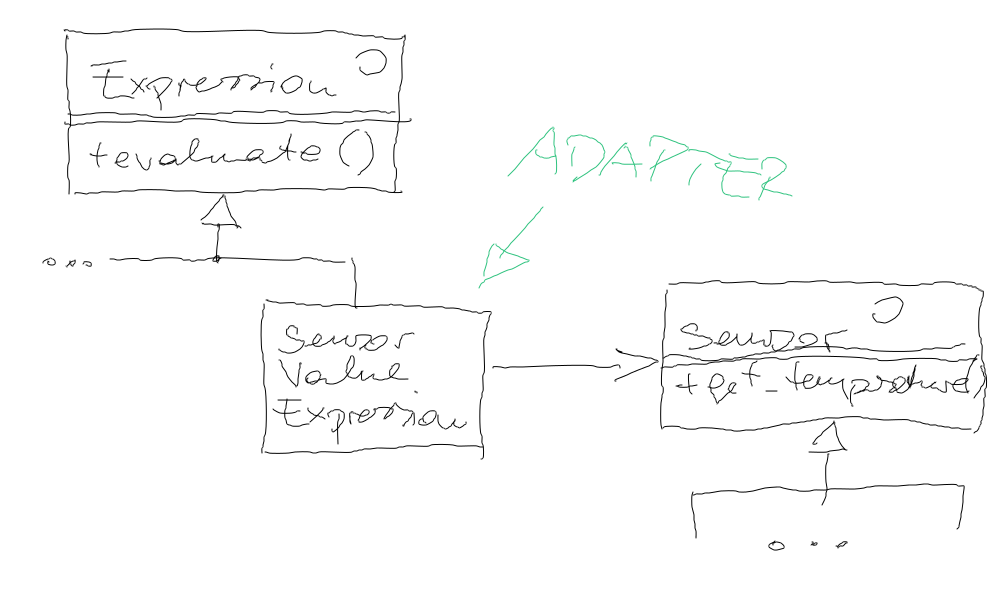# Exercise: Interpreter Combined With Adapter (And A Proxy)¶

## Motivation¶

In Interpreter we created a clas hierarchy to do arithmetic calculations, based on literals and variables - values, all in all.

The venerable `Sensor` hierarchy that we used in Exercise: Adapter and in Exercise: Proxy (Rounding Thermometer), for example, contains a number of classes (to be extended by real-world sensors one day), that also provide values.

Why not use a sensor as an `Expression`?## Requirement¶

In directory `programs/`, add an executable that is built from the following code:

```#include <sensor-random.h>
#include <sensor-round.h>
#include <expression.h>

#include <expression-sensorvalue.h>   // <--- to be created

#include <iostream>

int main()
{
RandomSensor s1(34.3, 40.6);
RandomSensor s2(35.1, 39.9);
RandomSensor s3(34.7, 38.8);

// sensors yield floating point numbers, while the expressions can
// only do integers. in order to avoid truncation during
// double->int conversion, we wrap rounding proxies around the raw
// sensors.
RoundingSensor r1(&s1);
RoundingSensor r2(&s2);
RoundingSensor r3(&s3);

// a specialized expression (to be implemented) that evaluates
// itself from sensors.
SensorValueExpression e1(&r1);    // <--- to be implemented
SensorValueExpression e2(&r2);    // <--- to be implemented
SensorValueExpression e3(&r3);    // <--- to be implemented

// use super-cool expression language on sensors to make
// measurements and calculate averages